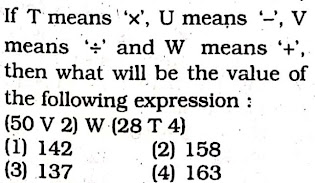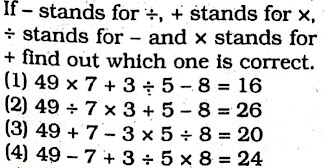## Symbols & Notations TYPE-I (i) Questions based on figures & mathematical operations

• Post author:
• Post category:Blog

### TYPE-I (i) Questions based on  figures & mathematical operations

Directions : In the following question you have to identify the correct response from the given premises stated according to following symbols :

1. If – ‘stands for division ‘+’ stands for subtraction,  stands for multiplication, ‘x’ stands for addition, then which one of the following equations is correct?

(1) 70 – 2 + 45 x 6 = 44

(2) 70 – 2 + 45×6 = 21

(3) 70-2+ 45 6 = 341

(4) 70 – 2 + 45 x 6 = 36

(SSC Combined Graduate Level Prelim Exam. 19.06.2011 (Ist Sitting)

2. If – stands for ‘ ‘ +’stands for ‘X’,’‘ for ‘-‘ and ‘x’ for ‘+’, which one of the following equations is correct?

(1) 30 – 6 + 5 x 4 2 = 27

(2) 30 + 6 – 5 4 x 2 = 30

(3) 30 x 6 5 – 4 + 2 = 32

(4) 30 6 x 5 + 4-2 = 40

(SSC Combined Graduate Level Tier-1 Exam. 26.06.2011 (Ist Sitting)

3.(SSC Multi-Tasking (Non-Technical). Staff Exam. 20.02:2011)4. (SSC Stenographer (Grade ‘C’ & ‘D’) Exam. 16.10.2011)5. (SSC Level Data Entry Operator & LDC Exam. 21.10.2012 (IstSitting)